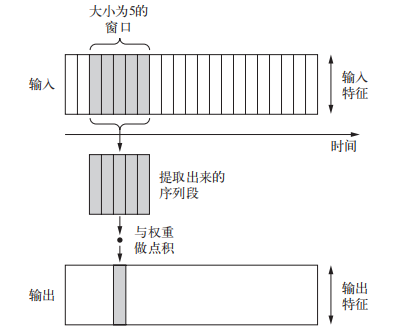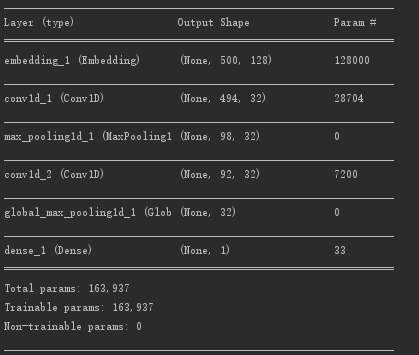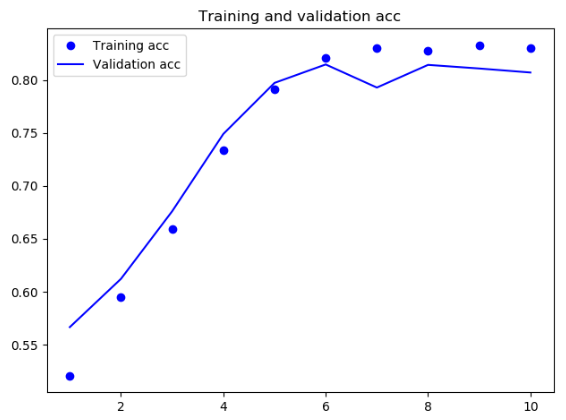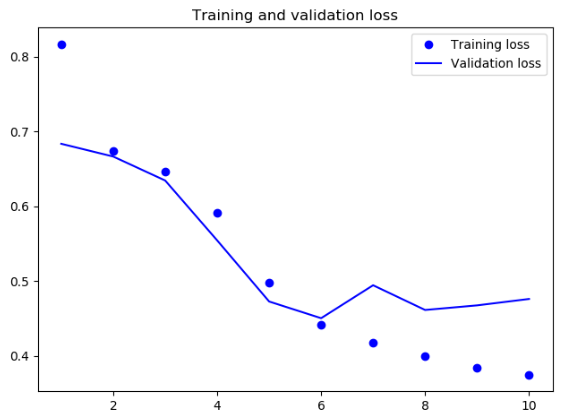# Deep learning with Python 学习笔记（7）Keras中的一维卷积神经网络是 Conv1D 层，它接收的输入形状是(samples, time, features)的三维张量，并返回类似形状的三维张量。卷积窗口是时间轴上的一维窗口（时间轴是输入张量的第二个轴）

Demo，使用网络架构为from keras.datasets import imdb
from keras.preprocessing import sequence
from keras.models import Sequential
from keras import layers
from keras.optimizers import RMSprop
import tools

max_features = 1000
max_len = 500

model = Sequential()
# 词嵌入，将人类的语言映射到几何空间中
# 对时域1D信号进行最大值池化
# 对于时间信号的全局最大池化

model.summary()
model.compile(optimizer=RMSprop(lr=1e-4),
loss='binary_crossentropy',
metrics=['acc'])
history = model.fit(x_train, y_train, epochs=10, batch_size=128, validation_split=0.2)
tools.draw_acc_and_loss(history)



tools.py

import matplotlib.pyplot as plt

# 绘制network fit history
def draw_acc_and_loss(history):
acc = history.history['acc']
val_acc = history.history['val_acc']
loss = history.history['loss']
val_loss = history.history['val_loss']
epochs = range(1, len(loss) + 1)
plt.figure()
plt.plot(epochs, acc, 'bo', label='Training acc')
plt.plot(epochs, val_acc, 'b', label='Validation acc')
plt.title('Training and validation acc')
plt.legend()
plt.show()

plt.plot(epochs, loss, 'bo', label='Training loss')
plt.plot(epochs, val_loss, 'b', label='Validation loss')
plt.title('Training and validation loss')
plt.legend()
plt.show()



AccLossposted @ 2018-12-26 22:16  范中豪  阅读(587)  评论(0编辑  收藏  举报